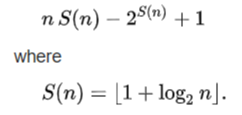# Sort Numbers Calculator | Sort Numbers in Ascending & Descending

With the help of our free online Sort Numbers Calculator, you can find any list of random numbers in an ascending and descending order in a blink of an eye. All you need to do is just give the input list in the below input field and click on the calculate button to make your calculations much easier & faster.

Sort Numbers: Sorting Numbers is a simple task to perform while doing lengthy calculations but if you need the result immediately then go with this amazing free online sort numbers tool. From this page, you can learn how to arrange a list in order with the help of Ascending & Descending Order Calculator ie., sort numbers calculator. Go through the further sections and get familiar with the order lists. Know more about the concept from here and make use of our online sort numbers calculator to sort the given list of numbers in a fraction of seconds.

## What is Sorting?

In mathematics & computer science, the sorting numbers are a sequence of numbers begun in 1950 by Hugo Steinhaus for the analysis of comparison sort algorithms. Also, Sorting is defined as arranging things in a certain order. There are two methods in sorting the list of numbers in mathematics ie., ascending order and descending order. The formula for n th sort number is given byThe sequence of numbers given by this formula (starting with n=1) is

0, 1, 3, 5, 8, 11, 14, 17, 21, 25, 29, 33, 37, 41, …

Let’s have a glance at the below section and learn how to sort the list in order (ascending & descending).

### How to Sort Numbers in Ascending & Descending Order list?

Ascending order is a sorting method where sort begins from the smallest value to the largest value or lowest to the highest number. In ascending sort the numbers will be arranged in smallest value to largest value, the letters and words will be sorted in alphabetical order like A to Z. In this form, the numbers are in increasing order. To represent this order of numbers, we use the ascending order symbol ‘<‘.

For instance, if you want to arrange the numbers from 10 to 15 in increasing order then the list will be sorted like this using < symbol is 10<11<12<13<14<15.

Descending Order is another sorting method. The sort of the numbers in descending order starts from the largest value to the smallest value. In this form, the numbers are in decreasing order. In descending sort, the numbers will be sorted from the bigger number to smaller ones and the alphabets will be sorted like Z to A. The symbol to represent in the descending order is ‘>’.

For instance, you want to arrange the list of 15 to 20 numbers in decreasing order then use the symbol and sort like this, 20>19>18>17>16>15.

Look at the below-solved example and get full knowledge on how to sort the list in ascending and descending order

Example:

How do you arrange the list in order? Look at the set of numbers 67, 898, 567, 4785, 45, 12, 5.

Solution:

To sort numbers in increasing order, place them from lowest (first) to highest (last). Then this is called ascending sort numbers.

The given list arranged in increasing form can be written as 5, 12, 45, 67, 567, 898, 4785.

To sort numbers in decreasing order, place them from highest (first) to lowest (last). This is known as sorting numbers in descending order.

The given list arranged in decreasing form can be written as 4785, 898, 567, 67, 45, 12, 5.

### Instructions to follow while using the Sort Numbers Calculator

The directions to use the sort numbers calculator are as under:

1. Initially, you have to Enter the numbers separated by a comma in the input field or Copy and paste the given list in the input field.
2. Now, tap on the Arrow button located right next to the input box in the calculator.
3. At last, the calculator displays the sort list in ascending and descending order below the keyboard on the screen.

Complete your maths assignments without any stress or mistakes by making use of free math calculators provided on onlinecalculator.guru

### FAQs on Sorting Numbers Calculator

1. How do you Sort Numbers in Mathematics?

First, consider the given list and then arrange in ascending sort or descending sort as per your requirement. To do that first take the smallest value and move with the next largest value in the list and make it sort list in ascending order and for descending sort numbers do the process in vice versa i.e, from large to a small value.

2. Can I find the Ascending and descending order list in Sort Numbers Calculator?

Yes, our free online sort numbers calculator provides the ascending and descending order list as an output in a fraction of seconds.

3. Arrange the list in order for the given set of numbers like 67, 8, 94, 25?

In the ascending sort list, the numbers are arranged like this 8,25,67,94.

In the descending sort list, the numbers are arranged like this 94,67,25,8.

4. Where can I learn about Sorting Numbers?

You can get complete knowledge about the concept of the sorting numbers from our page.